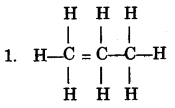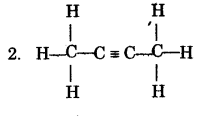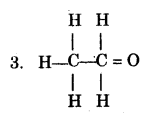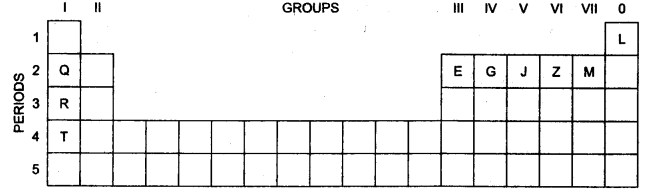### ICSE Class 10 Chemistry Previous Year Paper 2016# ICSE CLASS 10 CHEMISTRY PREVIOUS YEAR PAPER 2016

#### ICSE Class 10th chemistry Previous year paper 2016 Time: 2 hrs.                                                            Max. Marks: 80

• Answers to this Paper must be written on the paper provided separately.
• You will not be allowed 10 write during the first 15 minutes. This time is to be spent in reading the Question Paper.
• The time given at the head of this paper is the time allowed for writing the answers.
• Section I is compulsory. Attempt any four questions from section II.
• The intended marks for questions or parts of questions are given in brackets [ ]

SECTION I (40 Marks)
Attempt all questions from this Section

Question 1
(i) Fill in the blanks with the choices given in brackets                                                                                                         (a) Metals are good                        (oxidizing agents / reducing agents) because they are electron                       (acceptors / donors).
(b) Electrovalent compounds have                       (high / low) melting points.
(c) Higher the pH value of a solution, the more                       (acidic / alkaline) it is. (iv)                        (AgCl / PbCl2), a white precipitate is soluble in excess NH4OH.
(d) Conversion of ethane to ethane is an example of                       (hydration /hydrogenation).

(ii) Choose the correct answer from the options given below  (a) An element with the atomic number 19 will most likely combine chemically with the element whose atomic number is:
(A) 17
(B) 11
(C) 18
(D) 20
(b) The ratio between the number of molecules in 2g of hydrogen and 32g of oxygen is:
(A) 1: 2
(B) 1:0.01
(C) 1:1
(D) 0.01:1 [Given that H = 1, O = 16] (c) The two main metals in Bronze are:
(A) Copper and zinc
(C) Copper and nickel
(D) Copper and tin
(d) The particles present in strong electrolytes are:
(A) Only molecules
(B) Mainly ions
(C) Ions and molecules
(D) Only atoms
(e) The aim of the Fountain experiment is to prove that:
(A) HCl turns blue litmus red
(B) HCl is denser than air
(C) HCl is highly soluble in water
(D) HCl fumes in moist air
(iii) Write balanced chemical equations for each of the following: (a) Action of warm water on AIN.
(b) Action of hot and concentrated Nitric acid on copper.
(c) Action of Hydrochloric acid on sodium bicarbonate.
(d) Action of dilute Sulphuric acid on Sodium Sulphite.
(e) Preparation of ethanol from Ethyl Chloride.
(iv) State your observations when:                                                                                                                                       

(a) Dilute Hydrochloric acid is added to Lead nitrate solution and the mixture is heated.
(b) Barium chloride solution is mixed with Sodium Sulphate Solution.
(c) Concentrated Sulphuric acid is added to Sugar Crystals.
(d) Dilute Hydrochloric acid is added to Copper carbonate.
(e) Dilute Hydrochloric acid is added to Sodium thiosulphate.
(v) Identify the term / substance in each of the following:                                                                                               

(a) The tendency of an atom to attract electrons to itself when combined in a compound.
(b) The method used to separate ore from gangue by preferential wetting.
(c) The catalyst used in the conversion of ethyne to ethane.
(d) The type of reactions alkenes undergo.
(e) The electrons present in the outermost shell of an atom.
(vi)                                                                                                                                                                                                

(i) A gas of mass 32 gms has a volume of 20 litres at S.T.P. Calculate the gram molecular weight of the gas
(ii) How much Calcium oxide is formed when 82g of calcium nitrate is heated? Also find the volume of nitrogen dioxide evolved
begin{aligned}&2mathrm{Ca}left(mathrm{NO}_{3}right)_{2}rightarrow2mathrm{CaO}+4 mathrm{NO}_{2}+mathrm{O}_{2} \&(mathrm{Ca}=40,mathrm{~N}=14,0=16)end{aligned}
(vii) Match the salts given in Column I with their method of preparation given in Column II :                                   

$begin{array}{|l|l|}hline {text { Column I }}&{text{ColumnII}}\hlinetext{(i)}mathrm{Pb}left(mathrm{NO}_{3}right)_{2}text{from}mathrm{PbO} & text { A) Simple displacement } \text { (ii) } mathrm{MgCl}_{2} text{from}mathrm{Mg}&text { B) Titration } \text { (iii) } mathrm{FeCl}_{3} text{from}mathrm{Fe}&text { C) Neutralization } \text { (iv) } mathrm{NaNO}_{3} text {from}mathrm{NaOH}& text { D) Precipitation } \text { (v) } mathrm{ZnCO}_{3} text {from ZnSO }_{4} &text { E) Combination } \hlineend{array}$
(viii) Write the IUPAC names of each of the following:(ii) Rewrite the following sentences by using the correct symbol > (greater than) or < (less than) in the blanks given:
1. The ionization potential of potassium is                       that of sodium.
2. The electronegativity of lodine is                        that of Chlorine.

SECTION II (40 Marks)
Attempt any four questions from this Section

Question 2
(a) Use the letters only written in the Periodic Table given below to answer the questions that follow:i. State the number of valence electrons in atom J.
ii. Which element shown forms ions with a single negative charge?
iii. Which metallic element is more reactive than R?
iv. Which element has its electrons arranged in four shells?
(b) Fill in the blanks by selecting the correct word from the brackets:                                                                          

i. If an element has a low ionization energy, then it is likely to be                        (metallic / non-metallic).
ii. If an element has seven electrons in its outermost shell, then it is likely to have the                       (largest / smallest) atomic size among all the elements in the same period.
(c) The following table shows the electronic configuration of the elements W, X, Y, Z: Element

 Element W X Y Z Electronic configurations 2, 8, 1 2, 8, 7 2, 5 1

Answer the following questions based on the table above:
i. What type of Bond is formed between:                                                                                                           

1. W and X                                         2. Y and Z
ii. What is the formula of the compound formed between:                                                                              

1. X and Z                                           2. W and X

Question 3
(a) Write a balanced chemical equation for the following:                                                                                                 

(i) Burning of ethane in plentiful supply of air.
(ii) Action of water on Calcium carbide.
(iii) Heating of Ethanol at 170°C in the presence of conc. Sulphuric acid
(b) Give the structural formulae of each of the following:                                                                                               

(i) 2-methyl propane.
(ii) Ethanoic acid
(iii) Butan – 2 -ol
(c) Equation for the reaction when compound A is bubbled through bromine dissolved in carbon tetrachloride is as follows:                                                                                                                                        

i. Draw the structure of A.
ii. State your observation during this reaction.
(d) Fill in the blanks using the appropriate words given below:                                                                                         

(Sulphur dioxide, nitrogen dioxide, Nitric oxide, Sulphuric acid)
(i) Cold, dilute nitric acid reacts with copper to give                       .
(ii) Hot, concentrated nitric acid reacts with sulphur to form                       .

Question 4
(a) Identify the gas evolved and give the chemical test in each of the following cases:                                           

(i) Dilute hydrochloric acid reacts with sodium sulphite.
(ii) Dilute hydrochloric acid reacts with iron (II) sulphide.
(b) State your observations when ammonium hydroxide solution is added drop by drop and then in excess to each of the following solutions:                                                                                                                 

(i) Copper sulphate solution.
(ii) Zinc sulphate solution.
(c) Write equations for the reactions taking place at the two electrodes (mentioning clearly the name of the electrode) during the electrolysis of:                                                                                                       

(i) Acidified copper sulphate solution with copper electrodes.
(ii) Molten lead bromide with inert electrodes.
(d)                                                                                                                                                                                              

(i) Name the product formed at the anode during the electrolysis of acidified water using platinum electrodes.
(ii) Name the metallic ions that should be present in the electrolyte when an article made of copper is to be electroplated with silver.

Question 5
(a) A gas cylinder contains 12 x 1024 molecules of oxygen gas.                                                                                       

If Avogadro’s number is 6 x 1023; Calculate:
(i) The mass of oxygen present in the cylinder.
(ii) The volume of oxygen at S.T.P. present in the cylinder. [O = 16] (b) A gaseous hydrocarbon contains 82.76% of carbon. Given that its vapour density is 29, find its molecular formula.[C=12,H=11]                                                                                                                                

(c) The equation $4mathrm{NH}_{3}+5mathrm{O}_{2}rightarrow4mathrm{NO}+6mathrm{H}_{2} mathrm{O}_{4}$, represents the catalytic oxidation of ammonia. If 100 cm3 of ammonia is used calculate the volume of oxygen required to oxidize the ammonia completely. (d) By drawing an electron dot diagram show the formation of Ammonium ion [Atomic No.: N = 7 and H = 1]                                                                                                                                                                               

Question 6
(a) Name the gas evolved when the following mixtures are heated:                                                                             

(i) Calcium hydroxide and Ammonium Chloride
(ii) Sodium Nitrite and Ammonium Chloride
(b) Write balanced chemical equations for each of the following:                                                                                    

(i) When excess of ammonia is treated with chlorine.
(ii) An equation to illustrate the reducing nature of ammonia.
(c) A, B, C and D summarize the properties of sulphuric acid depending on whether it is dilute
or concentrated.                                                                                                                                                                       

A = Typical acid property
B = Nonvolatile acid
C = Oxidizing agent
D = Dehydrating agent
Choose the property (A, B, C or D) depending on which is relevant to each of the following
(i) Preparation of Hydrogen chloride gas.
(ii) Preparation of Copper sulphate from copper oxide.
(iii) Action of conc. Sulphuric acid on Sulphur.
(d) Give reasons why:                                                                                                                                                               

(i) Sodium Chloride will conduct electricity only in fused or aqueous solution state.
(ii) In the electroplating of an article with silver, the electrolyte sodium argent cyanide solution is preferred over silver nitrate solution.
(iii) Although copper is a good conductor of electricity, it is a non-electrolyte.

Question 7
(a)
(i) Name the solution used to react with Bauxite as a first step in obtaining pure aluminium oxide, in the Baeyer’s process.                                                                                                                               

(ii) Write the equation for the reaction where the aluminium oxide for the electrolytic extraction of aluminium is obtained by heating aluminium hydroxide.
(iii) Name the compound added to pure alumina to lower the fusion temperature during the electrolytic reduction of alumina.
(iv) Write the equation for the reaction that occurs at the cathode during the extraction of aluminium by electrolysis.
(v) Explain why it is preferable to use a number of graphite electrodes as anode instead of a single electrode, during the above electrolysis.
(b) State what would you observe when:                                                                                                                               

(i) Washing Soda Crystals are exposed to the atmosphere.
(ii) The salt ferric chloride is exposed to the atmosphere.
(c) Identify the cations in each of the following case:                                                                                                      

(i) NaOH solution when added to the Solution (A) gives a reddish-brown precipitate.
(ii) NH4OH Solution when added to the Solution (B) gives white ppt which does not dissolve in excess.
(iii) NaOH Solution when added to Solution (C) gives white ppt which is insoluble in excess.

Privacy Settings